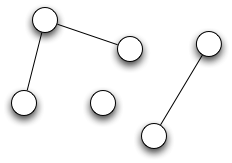Here we are interested in merging any two overlapping intervals into one interval. For example, if there are intervals 1 -> 4, 3 -> 10, 5 -> 7, 8 -> 16, then they all should be merged together to become one interval 1 -> 16 because there are two or more intervals that overlap.
1. 1 -> 4, 3 -> 10 overlap with each other, so they merge to give 1 -> 10.
2. Then we have the interval 5 -> 7. From previous step we got merged interval 1 -> 10. Since 5 -> 7 and 1 -> 10 are overlapping, they merge to give 1 -> 10.
3. Then 1 -> 10 and 8 -> 16 merge to yield 1 -> 16

Now let's take another example, 1 -> 4, 20 -> 22, 3 -> 10, 25 -> 35, 35 -> 40, 39 -> 61, 5 -> 7, 8 -> 16.
Here, 1 -> 4, 3 -> 10, 5 -> 7, 8 -> 16 merge together to yield 1 -> 16 ,
20 -> 22 has no overlap with any other intervals,
and 25 -> 35, 35 -> 40, 39 -> 61 overlap and merge to yield 25 -> 61
Output: 1 -> 16, 20 -> 22, 25 -> 61.

The above discussed problem can be solved in different ways as discussed below:

Let's define the Interval class as below:
``````
public class Interval {
public int start;
public int end;
}
```
```
1. ### Connected Components:

We can use Connected Components concept from graph theory to solve this problem. We can make a graph out of all the intervals, treating each interval as nodes. The overlapping intervals would make Connected Components. we then do graph traversal of each connected component, discover all overlapping intervals and merge them. make connected compo Algorithm:

• #### Build Graph:

We would represent graph as adjacency lists.
For each interval, we iterate over all other intervals and whenever we find an overlapping interval we add them in the adjacency list .
For any interval which has no overlapping intervals, we out an empty list as adjacency list.

• #### Find the Connected Components:

How do we find the connected components in the graph?If you try to visualize the graph now, the connected components will seem like island. The graph will be consisting of one or more disjoint sets or connected components. The graph in the picture above has 3 connected components. Each disjoint set consists of connected nodes. So we need to basically do a graph traversal of each of these disjoint sets (or, islands) or connected components and discover all the connected nodes in the connected components. So how can we achieve this? Simple, graph traversal like DFS or BFS.

For our implementation let's go with DFS.

• #### For each Connected ComponentsMerge all the intervals in the connected component into one.

To merge all the overlapping intervals from each connected component we just need to create one merged interval for which the start value will be the minimum of the start values of all the overlapping intervals in the currnt connected component, and the end value will be the maximum of the the end values of all the overlapping intervals in the current connected component.
``````
private int[] merge(List intervals) {
int[] res = intervals.get(0);
for (int i = 1; i < intervals.size(); i++) {
res = Math.min(res, intervals.get(i));
res = Math.max(res, intervals.get(i));
}
return res;

}
```
```

2. ### Sweep Line Algorithm:

We sort the intervals on the start value of the intervals and using LinkedList.
This approach is much more straight forward. We sort all the intervals on their start values, and then iterate over the sorted list of intervals. At every interval we look at the previous interval, and see if the start value is less than or equal to the end value of the previous interval. If yes, we merge these two intervals. If not we add this interval.

What data structure to use? Let's see. We need to have easy access to the last element in the data structure all the time. LinkedList would serve our purpose very well, as accessing tail is very convenient. We will go on adding any new interval, that needs to added, at the end of the linked list.

#### Instructor: